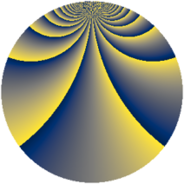# Properties

 Label 2304.3.biLevel $2304$ Weight $3$ Character orbit 2304.bi Rep. character $\chi_{2304}(353,\cdot)$ Character field $\Q(\zeta_{24})$ Dimension $1504$ Sturm bound $1152$

# Related objects

## Defining parameters

 Level: $$N$$ $$=$$ $$2304 = 2^{8} \cdot 3^{2}$$ Weight: $$k$$ $$=$$ $$3$$ Character orbit: $$[\chi]$$ $$=$$ 2304.bi (of order $$24$$ and degree $$8$$) Character conductor: $$\operatorname{cond}(\chi)$$ $$=$$ $$288$$ Character field: $$\Q(\zeta_{24})$$ Sturm bound: $$1152$$

## Dimensions

The following table gives the dimensions of various subspaces of $$M_{3}(2304, [\chi])$$.

Total New Old
Modular forms 6272 1568 4704
Cusp forms 6016 1504 4512
Eisenstein series 256 64 192

## Trace form

 $$1504q + 24q^{5} - 16q^{9} + O(q^{10})$$ $$1504q + 24q^{5} - 16q^{9} + 8q^{13} + 16q^{21} - 8q^{25} + 24q^{29} - 32q^{33} + 32q^{37} - 24q^{41} + 16q^{45} - 16q^{57} + 8q^{61} - 48q^{65} + 16q^{69} - 32q^{73} + 24q^{77} + 208q^{85} - 56q^{93} - 16q^{97} + O(q^{100})$$

## Decomposition of $$S_{3}^{\mathrm{new}}(2304, [\chi])$$ into newform subspaces

The newforms in this space have not yet been added to the LMFDB.

## Decomposition of $$S_{3}^{\mathrm{old}}(2304, [\chi])$$ into lower level spaces

$$S_{3}^{\mathrm{old}}(2304, [\chi]) \cong$$ $$S_{3}^{\mathrm{new}}(288, [\chi])$$$$^{\oplus 4}$$$$\oplus$$$$S_{3}^{\mathrm{new}}(1152, [\chi])$$$$^{\oplus 2}$$